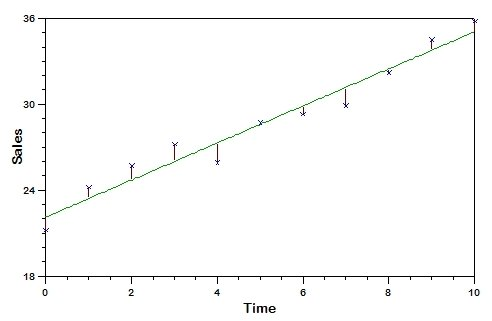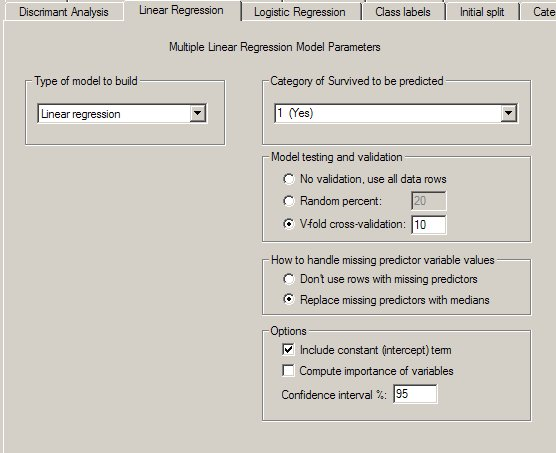# Linear Regression

Linear regression is the oldest and most widely used predictive model. The method of minimizing the sum of the squared errors to fit a straight line to a set of data points was published by Legendre in 1805 and by Gauss in 1809. The term “least squares” is from Legendre’s term, moindres carrés. However, Gauss claimed that he had known the method since 1795. The term "regression" was coined in the nineteenth century to describe a biological phenomenon, namely that the progeny of exceptional individuals tend on average to be less exceptional than their parents and more like their more distant ancestors [from Wikipedia].

A linear regression model fits a linear function to a set of data points. The form of the function is:

Y = β0 + β1*X1 + β2*X2 + … + βn*Xn

Where Y is the target variable, X1, X2, … Xn are the predictor variables, and β1, β2, … βn are coefficients that multiply the predictor variables. β0 is a constant.

For example, a function relating the strength of a material to hardness might be:

Strength = -0.8453 + 0.58388*Hardness

If there is a single predictor variable (X1), then the function describes a straight line. If there are two predictor variables, then the function describes a plane. If there are n predictor variables, then the function describes an n-dimensional hyperplane. Here is a plot of a fitted plane with two predictor variables:If a perfect fit existed between the function and the actual data, the actual value of the target value for each record in the data file would exactly equal the predicted value. Typically, however, this is not the case, and the difference between the actual value of the target variable and its predicted value for a particular observation is the error of the estimate which is known as the "deviation” or "residual”. The following plot depicts the residuals as red vertical lines connecting the data points and the fitted line.The goal of regression analysis is to determine the values of the ß parameters that minimize the sum of the squared residual values for the set of observations. This is known as a "least squares” regression fit. It is also sometimes referred to as “ordinary least squares” (OLS) regression.

Since linear regression is restricted to fitting linear (straight line/plane) functions to data, it rarely works as well on real-world data as more general techniques such as neural networks which can model non-linear functions. However, linear regression has a number of strengths:

• Linear regression is the most widely used method, and it is well understood.
• Training a linear regression model is usually much faster than methods such as neural networks.
• Linear regression models are simple and require minimum memory to implement, so they work well on embedded controllers that have limited memory space.
• By examining the magnitude and sign of the regression coefficients (β) you can infer how predictor variables affect the target outcome.

Linear regression is best suited for analyses with a continuous target variable, but DTREG also can create linear regression models to perform classification with a categorical target variable. When the target variable has two categories, a function is created to predict 1 for one of the categories and 0 for the other. If the target variable has more than two categories, DTREG creates a separate linear regression function for each category. The functions are trained to generate 1 if the category they are modeling is true and 0 for any other category.

If there are categorical predictor variables, DTREG generates a separate predictor variable for each category. A created predictor-category variable has the value 1 if the predictor variable has the category it represents and 0 if the predictor variable has any other category. If a categorical predictor variable has n categories, then (n-1) dummy variables are generated. Each generated variable has the value 1 if the variable’s value matches its associated category. All generated variables have the value 0 if the value of the predictor variable matches the remaining category. For example, if predictor variable TicketClass has three categories, FirstClass, Tourist and SuperSaver, then DTREG will arbitrarily select two of the categories for generated variables; let’s assume it selects FirstClass and Tourist. Then if the value of TicketClass is FirstClass, the generated variables would have the values: FirstClass=1, Tourist=0. If TicketClass was Tourist, then the generated variables would have the values: FirstClass=0, Tourist=1. And if TicketClass was SuperSaver, then the generated variables would have the values: FirstClass=0, Tourist=0.

Several computational algorithms can be used to perform linear regression. DTREG uses Singular Value Decomposition (SVD) which is robust and less sensitive to predictor variables that are nearly codependent.

Output Generated for Linear Regression

In addition to statistics measuring how well the function fits the data, DTREG generates a table showing the computed β coefficient values.

-------------- Computed Coefficient (Beta) Values -------------- Variable Coefficient Std. Error t Prob(t) 95% Confidence Interval -------- ------------- ------------ --------- --------- ------------ ------------ Hardness 0.583884 0.016 36.40 < 0.00001 0.5508 0.6169 Constant -0.845341 1.106 -0.76 0.45203 -3.124 1.434

A line is displayed showing the computed β coefficient for each predictor variable. If a constant (β0) is included in the equation, the last line shows the value of “Constant”.

Using the information in this table, we conclude that the function is:

Strength = -0.845341 + 0.583884*Hardness

In addition to the coefficient value, the standard error of the coefficient is displayed along with several other statistics:

t Statistic
The “t” statistic is computed by dividing the estimated value of the β coefficient by its standard error. This statistic is a measure of the likelihood that the actual value of the parameter is not zero. The larger the absolute value of t, the less likely that the actual value of the parameter could be zero. The t statistic probability is computed using a two-sided test.

Prob(t)
The “Prob(t)” value is the probability of obtaining the estimated value of the coefficient if the actual coefficient value is zero. The smaller the value of Prob(t), the more significant the coeficient and the less likely that the actual value is zero. For example, assume the estimated value of a parameter is 1.0 and its standard error is 0.7. Then the t value would be 1.43 (1.0/0.7). If the computed Prob(t) value was 0.05 then this indicates that there is only a 0.05 (5%) chance that the actual value of the parameter could be zero. If Prob(t) was 0.001 this indicates there is only 1 chance in 1000 that the parameter could be zero. If Prob(t) was 0.92 this indicates that there is a 92% probability that the actual value of the parameter could be zero; this implies that the term of the regression equation containing the parameter can be eliminated without significantly affecting the accuracy of the regression. One thing that can cause Prob(t) to be 1.00 (or near 1.00) is having redundant parameters. If at the end of an analysis several parameters have Prob(t) values of 1.00, check the function carefully to see if one or more of the parameters can be removed.

Confidence interval
The confidence interval shows the range of values for the computed coefficient that covers the actual coefficient value with the specified confidence. For example, the results above show a 95% confidence interval of 0.5508 to 0.6169 for the Hardness coefficient. This means that we are 95% confident that the true coefficient of Hardness falls in this range. You can set the percentage for the confidence interval on the Linear Regression Property Page.

Coefficients for categorical predictor variables

If some of the predictor variables have categorical values, then the table of computed coefficients has a line for each variable generated for categories. Here is an example:

-------------- Coefficient (Beta) Values for Survived = 1 (Yes) -------------- Variable Coefficient Std. Error t Prob(t) 95% Confidence Interval --------- ------------- ------------ --------- --------- ------------ ------------ Class Crew 0.131181 0.02164 6.06 < 0.00001 0.08875 0.1736 First 0.306734 0.02771 11.07 < 0.00001 0.2524 0.3611 Second 0.120654 0.02852 4.23 0.00002 0.06473 0.1766 Age Adult -0.181296 0.04097 -4.43 0.00001 -0.2616 -0.101 Sex Male -0.49068 0.02301 -21.33 < 0.00001 -0.5358 -0.4456 Constant 0.767591 0.04186 18.34 < 0.00001 0.6855 0.8497

Note that variables were generated for Crew, First and Second categories of Class. The variable generated for Age is 1 if Age=Adult and 0 otherwise. Similarly, there is a generated value for Sex that has the value 1 if Sex=Male and 0 otherwise.

DTREG Linear Regression Control Screen